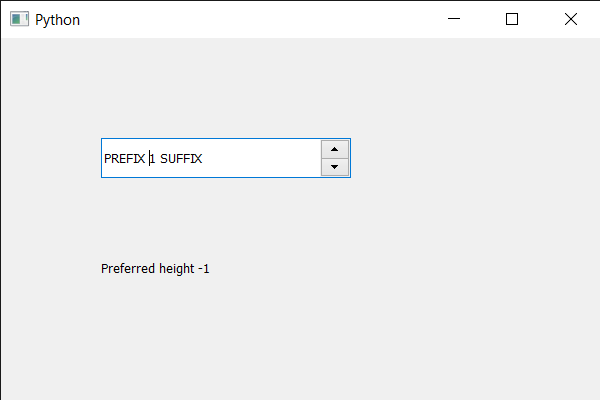# PyQt5 QSpinBox – How to get the preferred height for the given width

In this article we will see how we can get the preferred height of the spin box according to the width. Height depending on the width means that when ever the width changes height also changes. By default height don’t depends on the width.

Note : If spin box has a layout, the default implementation returns the layout’s preferred height. if there is no layout, the default implementation returns -1 indicating that the preferred height does not depend on the width.

In order to do this we use `heightForWidth` method with the spin box object.

Syntax : spin_box.heightForWidth(width)

Argument : It takes integer as argument

Return : It returns integer

Below is the implementation

 `# importing libraries ` `from` `PyQt5.QtWidgets ``import` `*`  `from` `PyQt5 ``import` `QtCore, QtGui ` `from` `PyQt5.QtGui ``import` `*`  `from` `PyQt5.QtCore ``import` `*`  `import` `sys ` ` `  ` `  `class` `Window(QMainWindow): ` ` `  `    ``def` `__init__(``self``): ` `        ``super``().__init__() ` ` `  `        ``# setting title ` `        ``self``.setWindowTitle(``"Python "``) ` ` `  `        ``# setting geometry ` `        ``self``.setGeometry(``100``, ``100``, ``600``, ``400``) ` ` `  `        ``# calling method ` `        ``self``.UiComponents() ` ` `  `        ``# showing all the widgets ` `        ``self``.show() ` ` `  `        ``# method for widgets ` `    ``def` `UiComponents(``self``): ` `        ``# creating spin box ` `        ``self``.spin ``=` `QSpinBox(``self``) ` ` `  `        ``# setting geometry to spin box ` `        ``self``.spin.setGeometry(``100``, ``100``, ``250``, ``40``) ` ` `  `        ``# setting range to the spin box ` `        ``self``.spin.setRange(``1``, ``999999``) ` ` `  `        ``# setting prefix to spin ` `        ``self``.spin.setPrefix(``"PREFIX "``) ` ` `  `        ``# setting suffix to spin ` `        ``self``.spin.setSuffix(``" SUFFIX"``) ` ` `  `        ``# creating a label ` `        ``label ``=` `QLabel(``self``) ` ` `  `        ``# making the label multi line ` `        ``label.setWordWrap(``True``) ` ` `  `        ``# setting geometry to the label ` `        ``label.setGeometry(``100``, ``200``, ``200``, ``60``) ` ` `  `        ``# getting preferred height of the spin box with current width ` `        ``value ``=` `self``.spin.heightForWidth(``250``) ` ` `  `        ``# setting text to the label ` `        ``label.setText(``"Preferred height "` `+` `str``(value)) ` ` `  ` `  `# create pyqt5 app ` `App ``=` `QApplication(sys.argv) ` ` `  `# create the instance of our Window ` `window ``=` `Window() ` ` `  `# start the app ` `sys.exit(App.``exec``()) `

Output :My Personal Notes arrow_drop_upCheck out this Author's contributed articles.

If you like GeeksforGeeks and would like to contribute, you can also write an article using contribute.geeksforgeeks.org or mail your article to contribute@geeksforgeeks.org. See your article appearing on the GeeksforGeeks main page and help other Geeks.

Please Improve this article if you find anything incorrect by clicking on the "Improve Article" button below.

Article Tags :

Be the First to upvote.

Please write to us at contribute@geeksforgeeks.org to report any issue with the above content.